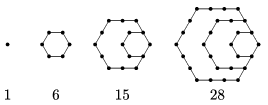Mathematical and Physical Journal
for High Schools
Issued by the MATFUND Foundation
 Already signed up? New to KöMaL?

#Problem K. 518. (November 2016)

K. 518. The numbers in the figures and further figures created in the same way are called hexagonal numbers. Without proof, find a formula for the $\displaystyle n$th hexagonal number, and use it to show that 2016 is a hexagonal number.(6 pont)

Deadline expired on December 12, 2016.

Sorry, the solution is available only in Hungarian. Google translation

Megoldás. „Emeletenként” nézve: $\displaystyle 1 = 1 \cdot 1$, $\displaystyle 6 = 3 \cdot 2$, $\displaystyle 15 = 5 \cdot 3$, $\displaystyle 28 = 7 \cdot 4$, az ötödik hatszögszám $\displaystyle 9 \cdot 5 = 45$, a hatodik pedig $\displaystyle 11 \cdot 6 = 66$ és így tovább. Az n. hatszögszám $\displaystyle (2n – 1) \cdot n$. Mivel $\displaystyle 2016 = 63 \cdot 32$, így a 2016 a 32. hatszögszám.

### Statistics:

 101 students sent a solution. 6 points: 84 students. 4 points: 6 students. 3 points: 2 students. 2 points: 5 students. 1 point: 1 student. 0 point: 3 students.

Problems in Mathematics of KöMaL, November 2016